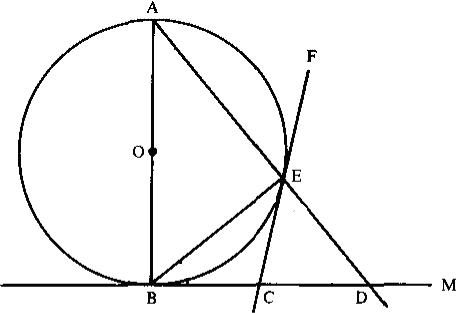$\def\bbN{{\mathbb N}} \def\bbQ{{\mathbb Q}} \def\bbR{{\mathbb R}} \def\bbZ{{\mathbb Z}}$
 © | Dror Bar-Natan: Classes: 2014-15: Math 475 - Problem Solving Seminar: (32) Next: Blackboards for Thursday February 26 Previous: Handout for February 24, "Divide into Cases" and "Work Backwards"

# Handout for February 26, "Divide into Cases", "Work Backwards", Pigeonhole

Reading. Sections 1.8 and 2.6 of Larson's textbook.

Next Quiz. Tuesday March 3 on these two sections and this handout.

Problem 1 (Larson's 2.5.11, modified).

1. Let $R_n$ denote the number of ways of placing $n$ non-attacking rooks on an $n\times n$ chessboard so that the resulting arrangement is symmetric about a $90^\circ$ clockwise rotation of the board about its centre. Compute $R_n$.
2. Let $S_n$ denote the number of ways of placing $n$ non-attacking rooks on an $n\times n$ chessboard so that the resulting arrangement is symmetric about the centre of the board. Compute $S_n$.
3. Let $T_n$ denote the number of ways of placing $n$ non-attacking rooks on an $n\times n$ chessboard so that the resulting arrangement is symmetric about both diagonals. Compute $T_n$.

Problem 2 (Larson's 2.5.13).

1. A derangement is a permutation $\sigma\in S_n$ such that for every $i$, $\sigma i\neq i$. Let $g_n$ be the number of derangements in $S_n$. Show that $g_1=0,\quad g_2=1,\quad g_n=(n-1)(g_{n-1}+g_{n-2}).$ Hint. A derangement interchanges $1$ with some other element, or not.
2. Let $f_n$ be the number of permutations in $S_n$ that have exactly one fixed point (namely, exactly one $i$ such that $\sigma i=i$). Show that $|f_n-g_n|=1$.Problem 3 (Larson's 1.8.1, modified).

1. Let $0<\alpha<\pi$. Show that $\frac{\sin\theta + \sin(\theta+\alpha)}{\cos\theta-\cos(\theta+\alpha)}$ is independent of $\theta$ for $0\leq\theta\leq\alpha$.
2. Can you find a geometric interpretation for this fact?

Problem 4 (Larson's 1.8.3). In the figure on the right, everything is as it seems: $O$ is the centre, $AB$ is a diameter, $CE$ and $BC$ are tangents, and all lines are straight. Show that $BC=CD$.

The Pigeonhole Principle. If 101 pigeons are put in 100 pigeonholes, at least one of the pigeonholes will be overcrowded. Or more generally,

If $kn+1$ objects are put in $n$ boxes, at least one of the boxes contains at least $k+1$ objects.

Problem 5 (Larson's 2.6.1). Given $n+1$ positive integers, none of which exceeds $2n$, show that one of these integers divides another of these integers.Problem 6 (Larson's 2.6.3, modified). The points on a $3\times 7$ rectangular grid are coloured $Q$ and $M$. Show that you can find a rectangle among these points, whose sides are parallel to the grid axes, and all of whose corners are coloured the same way.

Problem 7 (off topic, but fun). A rectangle is said to be "part whole" if the length of at least one of its sides is a whole number. Prove that if a rectangle $R$ can be partitioned into part whole subrectangles, then $R$ is part whole.

Hint. Consider $\int e^{2\pi i(x+y)}dx\,dy$. (!?!!)

New! I just learned of an article that has 14 proofs of this result! Fourteen Proofs of a Result About Tiling a Rectangle by Stan Wagon, The American Mathematical Monthly 94-7 (1987) 601-617.

Problem 8 (Larson's 2.6.6). If 20 integers are chosen from within the elements of the arithmetic progression 1,4,7,...,100, show that you can find two of them whose sum is 104.

Problem 9 (Larson's 2.6.10, expanded).

1. Let $X$ be any real number. Prove that among the numbers $X,\ 2X,\ \ldots,\ (n-1)X$ there is one that differs from an integer by at most $1/n$.
2. Let $\alpha$ be an irrational number. Prove that the set $\{\{n\alpha\}\colon n\in\bbZ\}$ is dense in the unit interval $[0,1]$, where for a real number $x$, $\{x\}$ denotes its "fractional part" - the difference between $x$ and the largest integer $\lfloor x\rfloor$ smaller or equal to $x$.

Problem 10 (Larson's 2.6.11, abbreviated).

1. Prove that in any group of six people there are either three mutual friends or three mutual strangers.
2. Prove that if all the edges and diagonals of a 17-gon are each coloured red, green, or blue, then you can find a single-colour triangle.